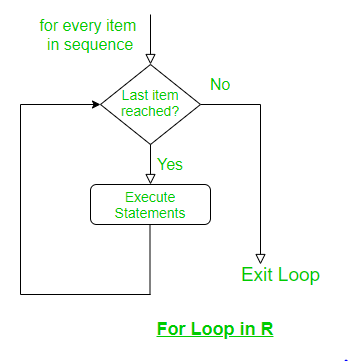Open in App
Not now

## Related Articles

• Write an Interview Experience

# For loop in R

• Difficulty Level : Easy
• Last Updated : 18 Oct, 2021

For loop in R Programming Language is useful to iterate over the elements of a list, dataframe, vector, matrix, or any other object. It means, the for loop can be used to execute a group of statements repeatedly depending upon the number of elements in the object. It is an entry controlled loop, in this loop the test condition is tested first, then the body of the loop is executed, the loop body would not be executed if the test condition is false.

## For loop in R Syntax:

```for (var in vector) {
statement(s)
}```

Here, var takes on each value of vector during the loop. In each iteration, the statements are evaluated.

## Flowchart of For loop in R:## R

 `# R Program to demonstrate``# the use of for loop``for ``(i ``in` `1: 4)``{``    ``print``(i ^ 2)``}`

Output:

``` 1
 4
 9
 16```

In the above example, we iterated over the range 1 to 4 which was our vector. Now there can be several variations of this general for loop. Instead of using a sequence 1:5, we can use the concatenate function as well.

## R

 `# R Program to demonstrate the use of``# for loop along with concatenate``for ``(i ``in` `c``(-8, 9, 11, 45))``{``    ``print``(i)``}`

Output:

``` -8
 9
 11
 45```

Instead of writing our vector inside the loop, we can also define it beforehand.

## R

 `# R Program to demonstrate the use of``# for loop with vector``x <- ``c``(-8, 9, 11, 45)``for ``(i ``in` `x)``{``    ``print``(i)``}`

Output:

``` -8
 9
 11
 45```

## Nested For-loop in R

R programming language allows using one loop inside another loop. In loop nesting, we can put any type of loop inside of any other type of loop. For example, a for loop can be inside a while loop or vice versa. The following section shows an example to illustrate the concept:

Example:

## R

 `# R Program to demonstrate the use of``# nested for loop``for ``(i ``in` `1:3)``{``    ``for ``(j ``in` `1:i)``    ``{``        ``print``(i * j)``    ``}``}`

Output:

``` 1
 2
 4
 3
 6
 9```

## Jump Statements in R

We use a jump statement in loops to terminate the loop at a particular iteration or to skip a particular iteration in the loop. The two most commonly used jump statements in loops are:

### Break Statement:

A break statement is a jump statement which is used to terminate the loop at a particular iteration. The program then continues with the next statement outside the loop(if any).

Example:

## R

 `# R Program to demonstrate the use of``# break in for loop``for ``(i ``in` `c``(3, 6, 23, 19, 0, 21))``{``    ``if ``(i == 0)``    ``{``        ``break``    ``}``   ``print``(i)``}``print``(``"Outside Loop"``)`

Output:

``` 3
 6
 23
 19
 Outside loop```

Here the loop exits as soon as zero is encountered.

### Next Statement

It discontinues a particular iteration and jumps to the next iteration. So when next is encountered, that iteration is discarded and the condition is checked again. If true, the next iteration is executed. Hence, the next statement is used to skip a particular iteration in the loop.

Example:

## R

 `# R Program to demonstrate the use of``# next in for loop``for ``(i ``in` `c``(3, 6, 23, 19, 0, 21))``{``    ``if ``(i == 0)``    ``{``        ``next``    ``}``    ``print``(i)``}``print``(``'Outside Loop'``)`

Output:

``` 3
 6
 23
 19
 21
 Outside loop```

Here as soon as zero is encountered, that iteration is discontinued and the condition is checked again. Since 21 is not equal to 0, it is printed. As we can conclude from the above two programs the basic difference between the two jump statements is that the break statement terminates the loop and the next statement skips a particular iteration of the loop.

My Personal Notes arrow_drop_up